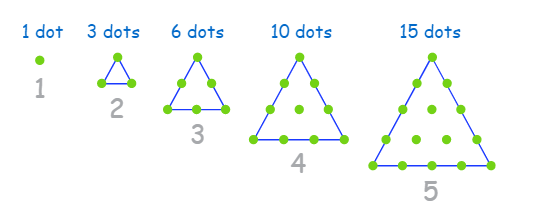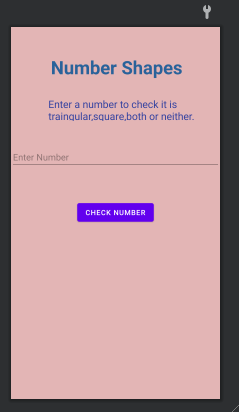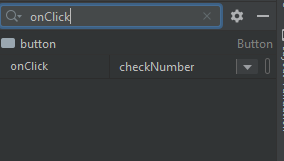Skip to content
Related Articles
How to Build a Number Shapes Android App in Android Studio?
• Last Updated : 27 May, 2021

Android is a vast field to learn and explore and to make this learning journey more interesting and productive we should keep trying new problems with new logic. So, today we are going to develop an application in android studio which tells us about Numbers that have shapes. We will be covering two types of numbers i.e. triangular and square. So, firstly let us know what actually they are:

### 1. Triangular Numbers

A number that can make a triangular dot pattern is known as a triangular number. For example 1, 3, 6, 10, 15 are triangular numbers.### 2. Square Numbers

A product of a number multiplied by itself is known as a square number. For example 1, 4, 9, 16, etc. In this application, we are going to whether a number is triangular, square, neither of them, or both of them.

### Step by Step Implementation

Step 1: Create a New Project

To create a new project in Android Studio please refer to How to Create/Start a New Project in Android Studio. Note that select Java as the programming language.

Step 2: Working with the activity_main.xml file

Navigate to the app > res > layout > activity_main.xml and add the below code to that file. Below is the code for the activity_main.xml file.

## XML

 ```<``androidx.constraintlayout.widget.ConstraintLayout``    ``xmlns:android``=``"http://schemas.android.com/apk/res/android"``    ``xmlns:app``=``"http://schemas.android.com/apk/res-auto"``    ``xmlns:tools``=``"http://schemas.android.com/tools"``    ``android:layout_width``=``"match_parent"``    ``android:layout_height``=``"match_parent"``    ``android:background``=``"#E3B5B5"``    ``tools:context``=``".MainActivity"``>` `    ``<``TextView``        ``android:id``=``"@+id/textView2"``        ``android:layout_width``=``"279dp"``        ``android:layout_height``=``"51dp"``        ``android:text``=``"Number Shapes"``        ``android:textColor``=``"#29629A"``        ``android:textSize``=``"36sp"``        ``android:textStyle``=``"bold"``        ``app:layout_constraintBottom_toBottomOf``=``"parent"``        ``app:layout_constraintHorizontal_bias``=``"0.592"``        ``app:layout_constraintLeft_toLeftOf``=``"parent"``        ``app:layout_constraintRight_toRightOf``=``"parent"``        ``app:layout_constraintTop_toTopOf``=``"parent"``        ``app:layout_constraintVertical_bias``=``"0.08"` `/>` `    ``<``TextView``        ``android:id``=``"@+id/textView"``        ``android:layout_width``=``"338dp"``        ``android:layout_height``=``"47dp"``        ``android:text``=``"Enter a number to check it is traingular,square,both or neither."``        ``android:textColor``=``"#303F9F"``        ``android:textSize``=``"20sp"``        ``app:layout_constraintBottom_toBottomOf``=``"parent"``        ``app:layout_constraintEnd_toEndOf``=``"parent"``        ``app:layout_constraintHorizontal_bias``=``"1.0"``        ``app:layout_constraintStart_toStartOf``=``"parent"``        ``app:layout_constraintTop_toBottomOf``=``"@+id/textView2"``        ``app:layout_constraintVertical_bias``=``"0.056"` `/>` `    ``<``EditText``        ``android:id``=``"@+id/editTextNumber"``        ``android:layout_width``=``"0dp"``        ``android:layout_height``=``"wrap_content"``        ``android:ems``=``"10"``        ``android:hint``=``"Enter Number"``        ``android:inputType``=``"number"``        ``app:layout_constraintBottom_toBottomOf``=``"parent"``        ``app:layout_constraintEnd_toEndOf``=``"parent"``        ``app:layout_constraintHorizontal_bias``=``"0.0"``        ``app:layout_constraintStart_toStartOf``=``"parent"``        ``app:layout_constraintTop_toBottomOf``=``"@+id/textView"``        ``app:layout_constraintVertical_bias``=``"0.097"` `/>` `    ``<``Button``        ``android:id``=``"@+id/button"``        ``android:layout_width``=``"wrap_content"``        ``android:layout_height``=``"wrap_content"``        ``android:onClick``=``"checkNumber"``        ``android:text``=``"Check Number"``        ``app:layout_constraintBottom_toBottomOf``=``"parent"``        ``app:layout_constraintEnd_toEndOf``=``"parent"``        ``app:layout_constraintStart_toStartOf``=``"parent"``        ``app:layout_constraintTop_toBottomOf``=``"@+id/editTextNumber"``        ``app:layout_constraintVertical_bias``=``"0.153"` `/>` ``

After writing this much code our UI looks like this:Do not forget to link the Check Number button with the function in java code. Use the following steps to do so:

Select the button “Check Number” and search onClick in its attributes.Write checkNumber there. Write logic of program in checkNumber() function in java file.

Step 3: Working with the MainActivity.java file

Go to the MainActivity.java file and refer to the following code. Below is the code for the MainActivity.java file. Comments are added inside the code to understand the code in more detail.

## Java

 `import` `android.os.Bundle;``import` `android.view.View;``import` `android.widget.EditText;``import` `android.widget.Toast;` `import` `androidx.appcompat.app.AppCompatActivity;` `public` `class` `MainActivity ``extends` `AppCompatActivity {` `    ``class` `number {``        ` `        ``int` `num;` `        ``public` `boolean` `isTraingular() {``            ``int` `a = ``1``;``            ``int` `traingular_number = ``1``;` `            ``while` `(traingular_number < num) {``                ``a++;``                ``traingular_number = traingular_number + a;``            ``}``            ``if` `(traingular_number == num) {``                ``return` `true``;``            ``} ``else``                ``return` `false``;``        ``}` `        ``public` `boolean` `isSquare() {``            ``double` `squareRoot = Math.sqrt(num);``            ``if` `(squareRoot == Math.floor(squareRoot))``                ``return` `true``;``            ``else` `return` `false``;``        ``}``    ``}``    ` `    ``public` `void` `checkNumber(View view) {``        ``String message = ``""``;``        ``EditText input_number = findViewById(R.id.editTextNumber);``        ``if` `(input_number.getText().toString().isEmpty()) {``            ``message = ``"Please enter a number!"``;``        ``} ``else` `{``            ``number mynum = ``new` `number();``            ``mynum.num = Integer.parseInt(input_number.getText().toString());` `            ``if` `(mynum.isSquare()) {``                ``if` `(mynum.isTraingular()) {``                    ``message = mynum.num + ``" is both a square and triangular number"``;``                ``} ``else` `{``                    ``message = mynum.num + ``" is a square number."``;``                ``}``            ``} ``else` `if` `(mynum.isTraingular()) {``                ``message = mynum.num + ``"is a triangular number."``;``            ``} ``else``                ``message = ``"It is neither traingular nor a square number"``;``        ``}``        ``Toast.makeText(``this``, message, Toast.LENGTH_SHORT).show();``    ``}``    ` `    ``@Override``    ``protected` `void` `onCreate(Bundle savedInstanceState) {``        ``super``.onCreate(savedInstanceState);``        ``setContentView(R.layout.activity_main);``    ``}``}`

After implementing all the above steps our application runs like this:

Hence we made a basic app that tells about number shapes and by making this application we learned about triangular and square numbers, how to link our button with java code, and prepare a simple UI.

Attention reader! Don’t stop learning now. Get hold of all the important Java Foundation and Collections concepts with the Fundamentals of Java and Java Collections Course at a student-friendly price and become industry ready. To complete your preparation from learning a language to DS Algo and many more,  please refer Complete Interview Preparation Course.

My Personal Notes arrow_drop_up